# LESSON 4_CIRCUIT (LECTURE).pptx

31 de Mar de 2023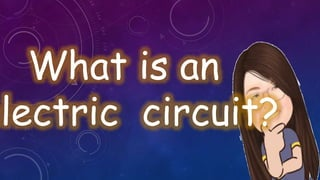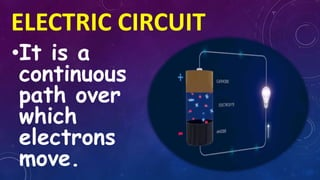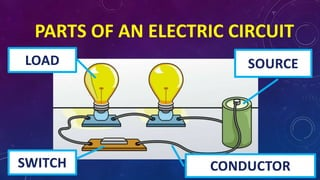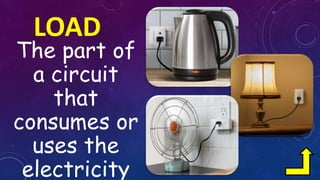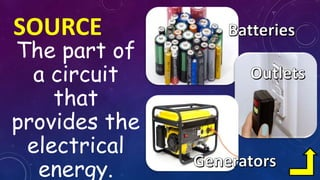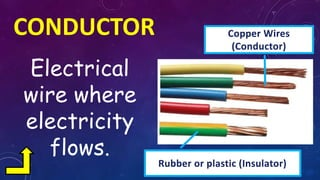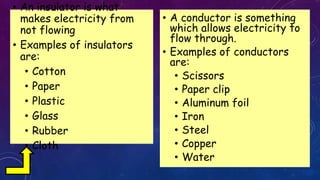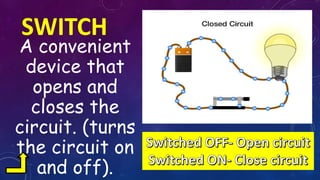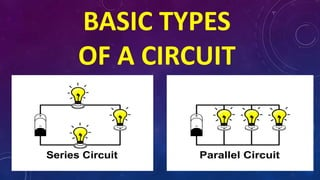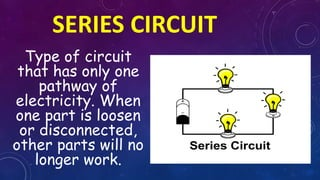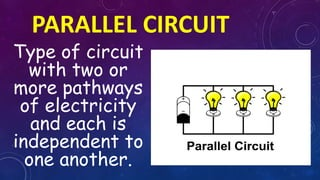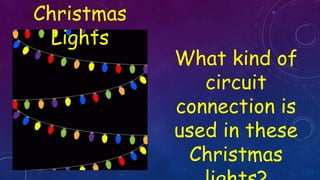1 de 12

### LESSON 4_CIRCUIT (LECTURE).pptx

1. What is an electric circuit?
2. ELECTRIC CIRCUIT •It is a continuous path over which electrons move.
3. PARTS OF AN ELECTRIC CIRCUIT LOAD SWITCH SOURCE CONDUCTOR
4. LOAD The part of a circuit that consumes or uses the electricity
5. SOURCE The part of a circuit that provides the electrical energy.
6. CONDUCTOR Electrical wire where electricity flows. Copper Wires (Conductor) Rubber or plastic (Insulator)
7. • An insulator is what makes electricity from not flowing • Examples of insulators are: • Cotton • Paper • Plastic • Glass • Rubber • Cloth • A conductor is something which allows electricity to flow through. • Examples of conductors are: • Scissors • Paper clip • Aluminum foil • Iron • Steel • Copper • Water
8. SWITCH A convenient device that opens and closes the circuit. (turns the circuit on and off).
9. BASIC TYPES OF A CIRCUIT
10. SERIES CIRCUIT Type of circuit that has only one pathway of electricity. When one part is loosen or disconnected, other parts will no longer work.
11. PARALLEL CIRCUIT Type of circuit with two or more pathways of electricity and each is independent to one another.
12. Christmas Lights What kind of circuit connection is used in these Christmas